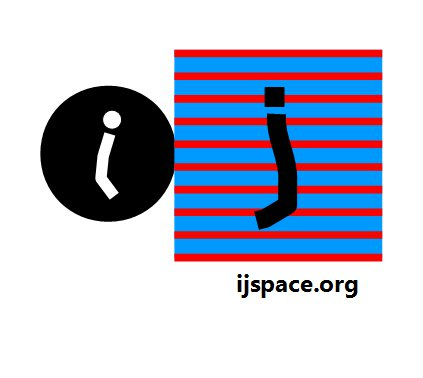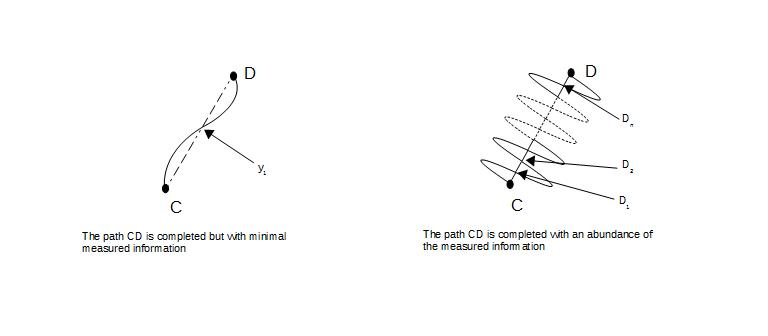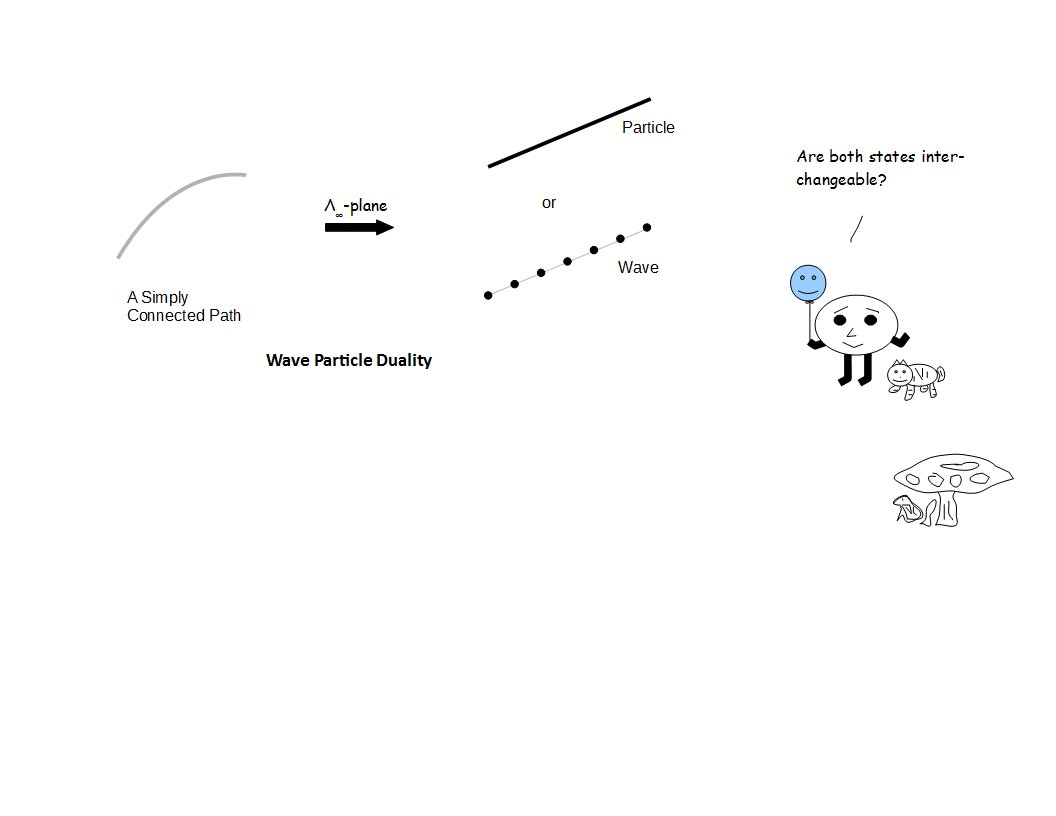Wave -Particle Duality 24th April 2016 (updated on 7th November 2018.) "Well, that's not quite right. I would say that I was absolutely convinced of the possibility of our making a uranium engine, but I never thought we would make a bomb, and at the bottom of my heart I was really glad that it was to be an engine and not a bomb. I must admit that."  - Heisenberg, Farm House Transcripts www.aip.org accessed  7Feb 2016.   "His career gives us confidence that the Renaissance ideal of a free and widely ranging knowledge will not vanish, however great the pressure of specialization." - Evans in introduction to Volterra's Theory of Functionals and of Integral and Integro-Differential Equations.   "Run you clever boy...and remember me." - Clara Oswald in Dr. Who. Let us consider an entropic path CD as measured by the macroscopic observer ObsM.  The path CD is not the path AB measured by ObsC (the observer with maximum efficiency), corresponding to ĥ.  The path CD represents more of a classical picture we are comfortable with.  Our objective is to measure all the information which is represented by this path CD.  The observers with different capacities will have measurements for the path CD with different information contents, as shown in the following figure*:Let us associate a variable to the length C-D2 and symbolize it with ƛ.  Then smaller the value of  the variable ƛ, greater is the information obtained from the path in j-space i.e.The above equation represents the equivalent of the De Broglie's relationship, in the discrete measurement space or j-space.  But still why this seemingly periodic motion around the path CD?     We have to realize that observer is not traveling in a straight line towards D, or it would have been just another single measurement or zero-entropy measurement scenario.  At the same time the observer can not move in a direction perpendicular to the path CD, else it will never arrive at D.  The wave-like character essentially is the observer's capacity to correct its course and not deviate from the path.  More accurate the measurement i.e. more information available to the observer, easier it is to correct course, hence smaller the value of ƛ**.      The path CD essentially defines the time axis in the observer's measurement metric.  For an observer with capacity v/c ~ 1, the time interval is [0, 1].  In Euclidean space we can make an assumption that time interval does not change for a range of observers with capacities in the range v/c ~ 1. In the traditional Euclidean space the information content is replaced by the momentum, and the above equation can be written in the form of the wavelength λ as,Thus higher the momentum, higher the information content extracted from interval [0, 1] and this we already know about our metric.  An example of this mechanism is a super-collider.     Above equation is the famous De Broglie equation.  However we have arrived it from our understanding of j-space.  The arguments are purely phenomenological.  Please note that we have not provided explanation for, why the proportionality constant is the Planck's constant h.  But we know that a resource can vary only in multiples of h and hence it makes sense to use the Planck's constant or a function linear in h, as the proportionality constant.     If the observer goes into a denser medium (assumed non-dispersive to simplify the argument) i.e. a medium which requires measurement of more information, the parameter λ must reduce and so will the quantity v/c,  quantity v/c (<<1).  But that does not mean the observer's ability to stay the course, has changed.  This must remain invariant or the measurement metric itself would change (i.e. VT-symmetry will be redefined and the measured value of the constant h would change).  The v/c ratio has gone down in the medium as well as the value of λ, only because now there is more information which needed to be measured completely.  We have to capture this fact i.e. the unchanged ability of the observer to stay the course, in a parameter.  Let us call it ν and we define it by using the concept of ratio as,What is being said here, is that the nature of the observer is an essential invariant in a measurement space and it is represented by ν.   Since the value of c is a fundamental constant for the macroscopic observer ObsM, we can assume c = 1j or simply c = 1, then the above relationship can be written as,Please note that the variable "v" the way it has been introduced here, is related to the "observer's capacity v/c" rather than the conventional "velocity", although we will eventually find it to be so in infinite momentum frame.      Similarly though "ν"  eventually would be found to be the equivalent to the concept of "frequency", we have not considered the concept of the time-period, or its relationship to the frequency in deriving the above relationship.  The advantage is that we have  stayed independent of the time-axis, which is a defining characteristic of the observer metric for ObsM.  Hence the relation, ν =  v/ƛ , being described here is more phenomenological in nature than conventional  "f = 1/time-period" formula.     It is important to realize that we can not use the familiar relationship between the frequency and the time-period without closing the measurement circuit or loop.  Closing the loop will require using the concept of angular frequency "ω" and at-least one irrational functional  such as "π".  Furthermore closing the loop is not possible in a pure discrete measurement space (Why?).     Finally we are able to associate an equivalent quantity called "momentum" in the observer's measurement metric to the observer's capacity to extract information. We are yet to determine the structure of the "momentum".     Thus to sum up, an observer in j-space has the following essential characteristics in a discrete measurement space:         1. Ability to extract information represented by variables "v/c" and ƛ,         2. Ability to correct its course represented by the variable ƛ,         3. Ability to stay the course represented by the variable ν. We can think of the variables "v/c", "ƛ", and "ν" as Skeleton parameters, whereas the equivalent parameters in Euclidean space "velocity", "momentum", "wavelength", "frequency" as Wrap parameters.  The Wrap parameters will have the dependence on "time" which represents the entropy inherent in the observer's measurements. We always want to develop our description using Skeleton parameters, which are based on phenomenological arguments.  The Wrap parameters are used to verify the Skeleton parameters, in the observers measurement metric space!.  Finally it is important to note that Euclidean space exists on the Λ∞-plane in the Anharmonic Coordinate representation. Is Euclidean space necessarily the only measurement space on the Λ∞-plane? ___________________ * We may notice that a wave like shape is being shown, which is a consequence of the Fourier's theorem. It could have been any signal with periodicity corresponding to ƛ. ** Can we think of an application for the case where ƛ is large yet the path CD is completed? Think deep and communicate. ! An example is the value of the fine-structure constant α = function(e1-q/ (π/2 + ε)): skeleton , and  α = e2/ħc : wrap representations. Previous Blogs:   Nutshell-2015 Chiral Symmetry Sigma-z and I Spin Matrices Rationale behind Irrational Numbers The Ubiquitous z-Axis Majorana ZFC Axioms Set Theory Nutshell-2014 Knots in j-Space Supercolliders Force Riemann Hypothesis Andromeda Nebula Infinite Fulcrum Cauchy and Gaussian Distributions Discrete Space, b-Field & Lower Mass Bound Incompleteness II The Supersymmetry The Cat in Box The Initial State and Symmetries Incompleteness I Discrete Measurement Space The Frog in Well Visual Complex Analysis The Einstein Theory of RelativityNope! Waves are approximation at best, to the particle which is essentially a topological state. Waves are wraps (t-axis exists during measurements, ObsM) while particles are skeletons (no time-axis, Obsc).

Best viewed with Internet Explorer